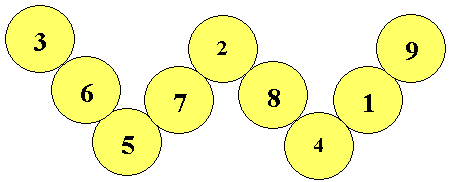#### You may also like### Instant Insanity

Given the nets of 4 cubes with the faces coloured in 4 colours, build a tower so that on each vertical wall no colour is repeated, that is all 4 colours appear.### Tree Graphs

A connected graph is a graph in which we can get from any vertex to any other by travelling along the edges. A tree is a connected graph with no closed circuits (or loops. Prove that every tree has exactly one more vertex than it has edges.### Magic Caterpillars

Label the joints and legs of these graph theory caterpillars so that the vertex sums are all equal.

# W Mates

##### Age 16 to 18 Challenge Level:

Here is one of the magic labellings of the W shape from Magic W problem with a magic total of $14$ on each of the four lines making the W shape.Prove that for every labelling with a magic total $T$ there is a corresponding labelling with a magic total $30-T$.

Find the values of $T$ for which magic labellings exist and show that there are a total of $12$ magic labellings altogether.

Note that two labellings are considered to be the same if they are reflections of each other or if the two numbers at the ends of the outside legs are interchanged.